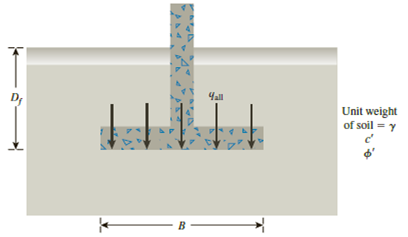Chapter 16, Problem 16.6PPrinciples of Geotechnical Enginee...

9th Edition
Braja M. Das + 1 other
ISBN: 9781305970939

Solutions

Chapter
SectionPrinciples of Geotechnical Enginee...

9th Edition
Braja M. Das + 1 other
ISBN: 9781305970939
Textbook Problem

Redo Problem 16.2 using the modified general ultimate bearing capacity Eq. (16.31).16.1 A continuous footing is shown in Figure 16.17. Using Terzaghi’s bearing capacity factors, determine the gross allowable load per unit area (qall) that the footing can carry. Assume general shear failure. Given: γ = 19 kN/m3, c′ = 31kN/m2, ϕ ′ = 28 ° , Df = 1.5 m, B = 2 m, and factor of safety = 3.5.Figure 16.176.2 Refer to Problem 16.1. If a square footing with dimension 2 m × 2 m is used instead of the wall footing, what would be the allowable bearing capacity?

To determine

Find the allowable bearing capacity using modified general ultimate bearing capacity.

Explanation

Given information:

The unit weight of the soil γ is 19kN/m3.

The value of cohesion c is 31kN/m2.

The soil friction angle ϕ is 28°.

The depth of foundation Df is 1.5 m.

The width of footing B is 2 m.

The factor of safety Fs is 3.5.

The size of the footing is (2m×2m).

Calculation:

Determine the shape factor λcs using the relation.

λcs=1+(BL)(NqNc)

Here, Nq is the contribution of surcharge, Nc is the contribution of cohesion,

Refer Table 16.2, “Bearing-capacity factors Nc, Nq, and Nγ” in the textbook.

Take the Nc as 25.80, Nq as 14.72, and Nγ as 16.72 for the ϕ value of 28°.

Substitute 2 m for B, 2 m for L, 14.72 for Nq, and 25.80 for Nc.

λcs=1+(22)(14.7225.80)=1.57

Determine the depth factor λcd using the relation.

λcd=1+0.4(DfB)

Substitute 1.5 m for Df and 2 m for B.

λcd=1+0.4(1.52)=1.3

Determine the shape factor λqs using the relation.

λqs=1+(BL)tanϕ

Substitute 2 m for B, 2 m for L, and 28° for ϕ.

λqs=1+(22)tan28°=1.53

Determine the depth factor λqd using the relation.

λqd=1+2tanϕ(1sinϕ)2(DfB)

Substitute 28° for ϕ, 1.5 m for Df, and 2 m for B.

λqd=1+2tan28°(1sin28°)2(1.52)=1.224

Determine the depth factor λγs using the relation.

λγs=10.4(BL)

Substitute 2 m for B and 2 m for L.

λγs=10.4(22)=0

Still sussing out bartleby?

Check out a sample textbook solution.

See a sample solution

The Solution to Your Study Problems

Bartleby provides explanations to thousands of textbook problems written by our experts, many with advanced degrees!

Get Started

Define the term big data.

Principles of Information Systems (MindTap Course List)

What role does a Linux distributor play?

Fundamentals of Information Systems

A workpiece offset is the distance from __________ to _________.

Precision Machining Technology (MindTap Course List)

What is a single sign on account? PIN stands for ___.

Enhanced Discovering Computers 2017 (Shelly Cashman Series) (MindTap Course List)